# The cylinder 2

Find the volume and the lateral area of a cylinder of height 12 inches and a base radius of 4 inches.

Correct result:

V =  603.1858 inch3
S2 =  301.5929 inch2

#### Solution: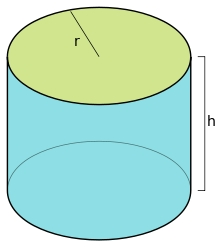We would be pleased if you find an error in the word problem, spelling mistakes, or inaccuracies and send it to us. Thank you!Tips to related online calculators
Tip: Our volume units converter will help you with the conversion of volume units.

#### You need to know the following knowledge to solve this word math problem:

We encourage you to watch this tutorial video on this math problem:

## Next similar math problems:

• KitchenKitchen roller has a diameter 70 mm and width of 359 mm. How many square millimeters roll on one turn?
• Cube volumeThe cube has a surface of 384 cm2. Calculate its volume.
• Total displacementCalculate total displacement of the 4-cylinder engine with the diameter of the piston bore B = 6.6 cm and stroke S=2.4 cm of the piston. Help: the crankshaft makes one revolution while the piston moves from the top of the cylinder to the bottom and back t
• Roller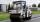Roller has a diameter of 0.96 m and a width 169 cm. How many m2 of road level when he turns 42-times?
• Reservoir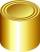6 m long reservoir has a diameter of 2.2 m. What is its surface area in square meters?
• Cone area and sideCalculate the surface area and volume of a rotating cone with a height of 1.25 dm and 17,8dm side.
• Water wellDrilled well has a depth 20 meters and 0.1 meters radius. How many liters of water can fit into the well?
• Sawdust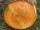How many cubic centimeters of wood sawdust is created by cut the tree trunk with a diameter of 66 cm and when the gap width is 5 mm?
• Giant coin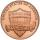From coinage metal was produced giant coin and was applied so much metal, such as production of 10 million actual coins. What has this giant coin diameter and thickness, if the ratio of diameter to thickness is the same as a real coin, which has a diamete
• Cylinder - A&VThe cylinder has a volume 1287. The base has a radius 10. What is the area of surface of the cylinder?
• Garden pondConcrete garden pond has bottom shape of a semicircle with a diameter 1.7 m and is 79 cm deep. Daddy wants make it surface. How many liters of water is in pond if watel level is 28 cm?
• The cylinder baseThe cylinder with a base of 8 dm2 has a volume of 120 liters. From a cylinder fully filled with water, 40 liters of water was removed. At what height from the bottom /with precision to dm/ is the water level?
• Rain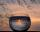How many mm of water rained the roof space 75 m2 if the empty barrel with a radius of 8 dm and height 1.2 m filled to 75% its capacity? :-)
• Rotary cylinder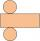The rotating cylinder has a surface area 69.08 cm2. The area of the shell is 62.8 cm 2. What is the diameter of the cylinder?
• Cylinder surface areaVolume of a cylinder whose height is equal to the radius of the base is 678.5 dm3. Calculate its surface area.
• Concrete pipe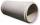Concrete pipe is cylindrical with an inner diameter 110 cm and outer 120 cm. Calculate the surface of the concrete pipe, if it is 9 m long.
• Cylinder - basicsCylinder with base radius r = 45 dm and height h=22 dm. Calculate: